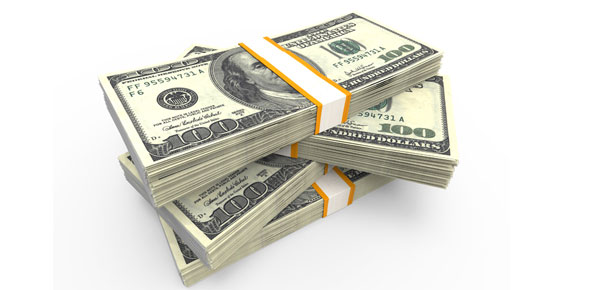# Money And Banking [ch. 4]

35 Questions | Total Attempts: 112SettingsUnderstanding Interest Rates

• 1.
Most people would prefer to receive \$100 on eyear form today than receive \$100 today because the present discounted value of a future cash flow is greater than the future cash flow
• A.

True

• B.

False

• 2.
A fixed-paymnet loan requires the borrower to make a single payment to the lender when the loan matures, and that single payment includes both the priciple and interest
• A.

True

• B.

False

• 3.
The coupon rate on a bond is the coupon divided by the face value (par) of the bond
• A.

True

• B.

False

• 4.
Yield to maturity is what economists mean when they use the term "interest rate."
• A.

True

• B.

False

• 5.
If a \$1,000 face value bond pays annual coupons of \$50, has two years to maturity, and has a yield to maturity of 7%, it will sell for \$963.84
• A.

True

• B.

False

• 6.
If the yield to maturity on a bond exceeds its coupon rate, the price of the bond will be above its face value
• A.

True

• B.

False

• 7.
The price of a bond and its yield to maturity are negative related
• A.

True

• B.

False

• 8.
The yield to maturity on a U.S. Treasury bill that sells for \$9,500 today, has a face value of \$10,000, and matures in one year, is 5%.
• A.

True

• B.

False

• 9.
If the nominal interest rate is 7% and expected inflation is 2%, then the real interest rate is 9%
• A.

True

• B.

False

• 10.
Current yield is a better estimate of yield to maturity for short-term bonds than for long-term bonds
• A.

True

• B.

False

• 11.
Current yield and yield to maturity on a perpetuity are the same
• A.

True

• B.

False

• 12.
If a bondholder pays \$1,000 for a 20-year bond that pays \$40 annual coupons, holds the bond for one year and than sells the bond for \$1,050, the rate of return for that year for the bondholder is 9%.
• A.

True

• B.

False

• 13.
A security that pays the holder \$500 five years from today is preferred to a security that pays the holder \$100 per year for the next five years
• A.

True

• B.

False

• 14.
If the interest rate falls the same amount for both short-term and long-term bonds, bondholders would prefer to be holding short-term bonds
• A.

True

• B.

False

• 15.
Borrowers have a greater desire to borrow when the nominal interest rate is 15% and the expected inflation rate is 13% than when the nominal interest rate is 6% and the expected inflation rate is 2%.
• A.

True

• B.

False

• 16.
The most accurate measure of interest rates is
• A.

The coupon rate

• B.

Yield to maturity

• C.

Current yield

• D.

Discounted present value

• 17.
If the interest rate is 5%, the present value of \$1,000 to be received five years from today is
• A.

\$783.53

• B.

\$866.66

• C.

\$952.38

• D.

\$1,000.00

• E.

\$1,050.00

• 18.
If a borrow must repay \$106.50 one year from today in order to receieve a simple loan of \$100 today, the simple interest on this loan is
• A.

65%

• B.

5.0%

• C.

6.1%

• D.

6.5%

• E.

None of the above

• 19.
You are in a car accident, and you receive an insurance settlemnt of \$5,000 per year for the next three years.  The first payment is to be received today.  The second payment is to be received one year from today, and the third payment two years from today.  If the interest rate is 6%, the present value of the insurance settlement is
• A.

\$15,000.00

• B.

%14,166.96

• C.

\$13,365.06

• D.

\$13,157.98

• 20.
Which of the following instruments pays the holder of the instrument a fixed interest payment every year until maturity, and then at maturity pays the holder the face value (principle) of the instrument?
• A.

Simple loan

• B.

Fixed-payment loan

• C.

Coupon bond

• D.

Discount bond

• 21.
A U.S. Treasury bill is an example of a
• A.

Simple loan

• B.

Fixed-payment loan

• C.

Coupon bond

• D.

Discount bond

• 22.
A coupon bond with a face value of \$1,000 that pays an annual coupon of \$100 has a coupon rate of
• A.

\$100

• B.

\$1,100

• C.

10%

• D.

9.1%

• E.

None of the above

• 23.
What is teh approximate yield to maturity on a coupon bond that matures one year from today, has a par value of \$1,000, pays an annual coupon of \$70, and whose price today is \$1,019.05
• A.

4%

• B.

5%

• C.

6%

• D.

7%

• E.

8%

• 24.
What price will al coupon bond sell for if it has two years to maturity, a coupon rate of 8%, a par value of \$1,000, and a yield to maturity of 12%?
• A.

\$920.00

• B.

\$924.74

• C.

\$932.40

• D.

\$1,035.71

• E.

\$1,120.00

• 25.
Which of the following bonds has the highest yield to maturity?
• A.

A 20-year, \$1,000 par, 5% coupon bond selling for \$900

• B.

A 20-year, \$1,000 par, 5% coupon bond selling for \$1,000

• C.

A 20-year, \$1,000 par, 5% coupon bond selling for \$1,100

• D.

There is not enough information to answer this question

Related TopicsBack to top